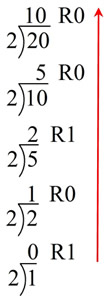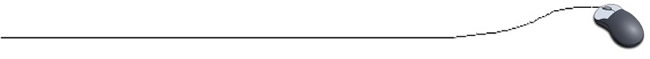Convert a number from any base to any other base:When converting from any number base to any other number base,
and neither of the bases is base 10
,
you must go via (through) base 10.

Example:  Convert 248 into base 2.

First, convert 248 into base 10.

 4 x 80 = 4   2 x 81 = 16 4 + 16 = 20

248 = 2010

Second, convert that answer into base 2.2010 = 101002